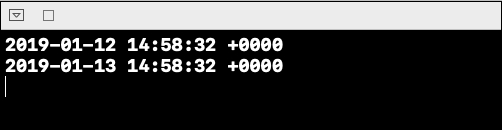# How add 1 day to Date in swift?

To 1 day to a date in swift we need to create a date first. Once that date is created we have to add specific days to it. In this example we’ll see how we can achieve the same.

Let’s create a date first, let it be today,

let today = Date()

Now to modify this date we’ll use the add function with negative value,

let modifiedDate = Calendar.current.date(byAdding: .day, value: 1, to: today)!

Now to see the difference between both the dates, let’s add print statement for both of these. Our complete code should now look like this.

let today = Date()
print(today)
let modifiedDate = Calendar.current.date(byAdding: .day, value: 1, to: today)!
print(modifiedDate)

When we run the above code on a playground simulator we get the following result, which shows exactly a difference of two hours in both the date, and the modified date.